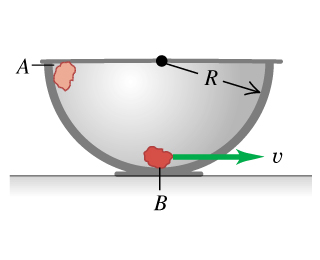# Problem: A small rock with mass 0.22 kg is released from rest at point exttip{A}{A}, which is at the top edge of a large, hemispherical bowl with radius exttip{R}{R} = 0.52 m (the figure ). Assume that the size of the rock is small compared to R, so that the rock can be treated as a particle, and assume that the rock slides rather than rolls. The work done by friction on the rock when it moves from point exttip{A}{A} to point exttip{B}{B} at the bottom of the bowl has magnitude 0.22 J.Between points exttip{A}{A} and exttip{B}{B}, how much work is done on the rock by the normal force?What is the speed of the rock as it reaches point exttip{B}{B}?Of the three forces acting on the rock as it slides down the bowl, which (if any) are constant and which are not? Explain.Just as the rock reaches point exttip{B}{B}, what is the normal force on it due to the bottom of the bowl?Between points exttip{A}{A} and exttip{B}{B}, how much work is done on the rock by gravity?

###### FREE Expert Solution
98% (304 ratings)
###### Problem Details

A small rock with mass 0.22 kg is released from rest at point , which is at the top edge of a large, hemispherical bowl with radius = 0.52 m (the figure). Assume that the size of the rock is small compared to R, so that the rock can be treated as a particle, and assume that the rock slides rather than rolls. The work done by friction on the rock when it moves from point to point at the bottom of the bowl has magnitude 0.22 J.

Between points and , how much work is done on the rock by the normal force?

What is the speed of the rock as it reaches point ?

Of the three forces acting on the rock as it slides down the bowl, which (if any) are constant and which are not? Explain.

Just as the rock reaches point , what is the normal force on it due to the bottom of the bowl?

Between points and , how much work is done on the rock by gravity?

Frequently Asked Questions

What scientific concept do you need to know in order to solve this problem?

Our tutors have indicated that to solve this problem you will need to apply the Energy with Non-Conservative Forces concept. You can view video lessons to learn Energy with Non-Conservative Forces. Or if you need more Energy with Non-Conservative Forces practice, you can also practice Energy with Non-Conservative Forces practice problems.